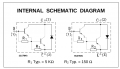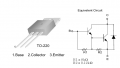# Emitter-Follower Amplifier Using A TIP120

#### elec_eng_55

Joined May 13, 2018
214
Hi Folks:

I am studying an Emitter-Follower Amplifier using a TIP120.
There are two things that I can't understand.

1) The Base voltage in the simulation and the real world
turns out to be 10.23 volts. If you use the voltage divider
calculation, it should be 11.51 volts. If you disconnect the
base of the darlington from the voltage divider, the voltage
divider does show 11.51 volts.

2) The output signal in the real world is riding on a negative
dc level instead of 0 volts. The first thing I thought of was
to replace the output coupling capacitor but that made no
difference.

I am perplexed. Any help would be appreciated.

Thanks,

David

#### ericgibbs

Joined Jan 29, 2010
13,650
hi 55,
E

#### Kjeldgaard

Joined Apr 7, 2016
453
I do not have an answer to all but question 1: It looks like a problem with the two internal base/emitter resistors.

Joined Mar 10, 2018
4,057Regards, Dana.

Last edited:

#### ebp

Joined Feb 8, 2018
2,332
The only possible way to get a base voltage of 11.51 V is if the gain of the darlington is infinite. It isn't. A quick look at the gain curves says it is around 1000 to 1200 at the probable collector current.

[EDIT] The average output voltage must be exactly zero. It cannot be otherwise. This does not mean that the midpoint between the peaks is zero volts.

•Oscar Mantovani

#### elec_eng_55

Joined May 13, 2018
214
hi 55,
E
LTS acc is now there.

#### Attachments

• 2 KB Views: 14

#### bertus

Joined Apr 5, 2008
21,549
Hello,

As @danadak told you, there are some internal resistors.
Even the values of the internal resistors can be different for other manufacturers.
ST shows this :Fairchild shows this :Always check the actual datasheet.

Bertus

•RichardO

#### ebp

Joined Feb 8, 2018
2,332
The internal resistors really don't need to be considered. All that is required to get an idea of whether the predicted voltage at the base is reasonable is to look at the datasheet and do some very simple approximate calculations.

Suppose the base is fixed at 11.5 volts (all numeric values in the following should be considered to be approximate)
• the emitter voltage would be at 10.2 volts due to the two Vbe drops between base and emitter
• the collector current would therefore be 10.2/56 = 180 mA
• the datasheet (Fig. 9 of ON Semi sheet) shows typical current gain of 1000 at that current at 25°C
• base current must therefore be 180 µA - we don't care whether it goes through the actual base of the transistor or through the resistors
• an "extra" 180 µA through 10k ohms is 1.8 volts (this is really over-simplifying because the network isn't properly solved, but it gives a crude approximation), so the base voltage is clearly going to be considerably less than 11.5 volts
• the original assumption that the base voltage would be determined only by the voltage divider, with zero base current, is clearly unreasonable
We don't have accurate numbers from this very simple analysis, but we do have confirmation that the original assumption is not reasonable because it ignores a current that is too large to be ignored. If the total bias network resistance were reduced by a factor of 10, clearly the base voltage would be considerably closer to the predicted 11.5 volts

•ebeowulf17

#### ericgibbs

Joined Jan 29, 2010
13,650
hi 55,
The only difference' I can see is in the TIP120 Model parameters, ie: R1= 10k and R2=1k as opposed to the values shown in post #4 ie: 8k and 120R
This sim shows what I see, which looks OK.
E

#### Attachments

•elec_eng_55

#### elec_eng_55

Joined May 13, 2018
214
Hi Folks:

I am studying an Emitter-Follower Amplifier using a TIP120.
There are two things that I can't understand.

1) The Base voltage in the simulation and the real world
turns out to be 10.23 volts. If you use the voltage divider
calculation, it should be 11.51 volts. If you disconnect the
base of the darlington from the voltage divider, the voltage
divider does show 11.51 volts.

2) The output signal in the real world is riding on a negative
dc level instead of 0 volts. The first thing I thought of was
to replace the output coupling capacitor but that made no
difference.

I am perplexed. Any help would be appreciated.

Thanks,

David
Thank you everyone!

#### ebeowulf17

Joined Aug 12, 2014
3,282
The internal resistors really don't need to be considered. All that is required to get an idea of whether the predicted voltage at the base is reasonable is to look at the datasheet and do some very simple approximate calculations.

Suppose the base is fixed at 11.5 volts (all numeric values in the following should be considered to be approximate)
• the emitter voltage would be at 10.2 volts due to the two Vbe drops between base and emitter
• the collector current would therefore be 10.2/56 = 180 mA
• the datasheet (Fig. 9 of ON Semi sheet) shows typical current gain of 1000 at that current at 25°C
• base current must therefore be 180 µA - we don't care whether it goes through the actual base of the transistor or through the resistors
• an "extra" 180 µA through 10k ohms is 1.8 volts (this is really over-simplifying because the network isn't properly solved, but it gives a crude approximation), so the base voltage is clearly going to be considerably less than 11.5 volts
• the original assumption that the base voltage would be determined only by the voltage divider, with zero base current, is clearly unreasonable
We don't have accurate numbers from this very simple analysis, but we do have confirmation that the original assumption is not reasonable because it ignores a current that is too large to be ignored. If the total bias network resistance were reduced by a factor of 10, clearly the base voltage would be considerably closer to the predicted 11.5 volts
Thanks for that! At first glance, I suspected it was current through the base(s) pulling voltage down, but didn't know how to analyze it. Your example was wonderful!

Last edited:

#### AnalogKid

Joined Aug 1, 2013
9,358
The only difference' I can see is in the TIP120 Model parameters, ie: R1= 10k and R2=1k as opposed to the values shown in post #4 ie: 8k and 120R
This sim shows what I see, which looks OK.
That is both excellent information and distressing news. "Never trust sim libraries" is something everyone learns the hard way, but the TIP series has been around for *decades*. Not good to see generic resistor values that are almost a full order of magnitude off reality.

If you want to see the real effects of the internal resistors, replace the TIP120 in the sim with a 2N2222, TIP31, and the correct resistor values in parallel.

ak

#### ebp

Joined Feb 8, 2018
2,332
The current ST datasheet shows R2 as typically 70 ohms. A TI datasheet form the early 1980s shows it as typically 150 ohms.

In the TS's original DC analysis, why are two values for the current in R1 shown? One value is many orders of magnitude small than the other. No other parameter gets such special treatment.

I should clarify that when I said "The internal resistors really don't need to be considered." what I meant is that the effects of those resistors are already rolled into the numeric and graphical current gain information in the datasheet. They most certainly must be considered for details of behavior, especially turn-off when the device is used as a switch and collector current at high temperature.

#### elec_eng_55

Joined May 13, 2018
214
I bread boarded the circuit. When I scoped the output it is a train of sine
waves but the string of waves jumps up and down. What the heck is that

#### AnalogKid

Joined Aug 1, 2013
9,358

#### elec_eng_55

Joined May 13, 2018
214
All test circuits work except the one using a TIP120. I am using a new proto board.and
a new TIP120. Still no dice.

#### Ylli

Joined Nov 13, 2015
1,053
This is simple enough. Take it off the proto board and 'dead-bug' wire it. That will eliminate any possible issue with your proto board.